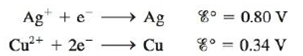Chapter 18, Problem 138CWP

Chapter
Section
Textbook Problem

# An electrochemical cell consists of a silver metal electrode immersed in a solution with [Ag+] = 1.00 M separated by a porous disk from a compartment with a copper metal electrode immersed in a solution of 10.00 M NH3 that also contains 2.4 × 10−3 M Cu(NH3)42+. The equilibrium between Cu2+ and NH3 is: Cu 2 + ( a q )   +   4 NH 3 ( a q )   ⇌ Cu ( N ​ H 3 ) 4 2 + ( a q ) K = 1.0 × 10 13 and the two cell half-reactions are:Assuming Ag+ is reduced, what is the cell potential at 25°C?

Interpretation Introduction

Interpretation:

An electrochemical cell consisting of Silver and Copper electrode dipped in Ammonia solution and its equilibrium constant is given. The value of cell potential at 25°C for the given cell is to be calculated.

Concept introduction:

The relationship between reduction potential and standard reduction potential value and activities of species present in an electrochemical cell at a given temperature is given by the Nernst equation.

The value of Ecell is calculated using Nernst formula,

E=E°(RTnF)ln(Q)

At room temperature, the above equation specifies as,

E=E°(0.0591n)log(Q)

To determine: The value of cell potential at 25°C for the given cell.

Explanation

Given,

The concentration of Ag+ is 1.00M .

The concentration of NH3 solution is 1.00M .

The concentration of NH3 solution is 1.00M .

The concentration of [Cu(NH3)4]2+ solution is 2.4×103M .

The value of the equilibrium constant is 1.0×1013

The equilibrium reaction is,

Cu2+(aq)+4NH3(aq)[Cu(NH3)4]2+(aq)

The reaction taking place at the cathode is,

Ag++eAgE°red=0.80V

The reaction taking place at the anode is,

CuCu2++2eE°OX=0.34V

Multiply reduction half-reaction with a coefficient of 2 and then add both the reactions as,

2Ag++2e2AgCuCu2++2e

The final equation is,

2Ag++Cu2Ag+Cu2+

The value of E°cell is given as,

E°cell=E°ox+E°red

Where,

• E°ox is the oxidation potential of the electrode.
• E°red is the reduction potential of the electrode.

Substitute the values of E°ox and E°red in the above equation as,

E°cell=E°ox+E°red=0

### Still sussing out bartleby?

Check out a sample textbook solution.

See a sample solution

#### The Solution to Your Study Problems

Bartleby provides explanations to thousands of textbook problems written by our experts, many with advanced degrees!

Get Started